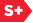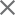### Proceedings Paper

Fast approximate Fourier transform via wavelets transform
Author(s): Haitao Guo; C. Sidney Burrus
Format Member Price Non-Member Price
PDF \$17.00 \$21.00

Paper Abstract

We propose an algorithm that uses the discrete wavelet transform as a tool to compute the discrete Fourier transform (DFT). The Cooley-Tukey FFT is shown to be a special case of the proposed algorithm when the wavelets in use are trivial. If no intermediate coefficients are dropped and no approximations are made, the proposed algorithm computes the exact results, and its computational complexity is on the same order of the FFT. The main advantage of the proposed algorithm is that the good time and frequency localization of wavelets can be exploited to approximate the Fourier transform for many classes of signals resulting in much less computation. Thus the new algorithm provides an efficient complexity vs accuracy tradeoff. When approximations are allowed, under certain sparsity conditions, the algorithm can achieve linear complexity. It has been shown that the thresholding of the wavelet coefficients has near optimal noise reduction property for many classes of signals. We show that for the same reason, the proposed algorithm also reduces the noise while doing the approximation. If we need to compute the DFT of noisy signals, the proposed algorithm not only can reduce the numerical complexity, but also can produce cleaner results. In summary, we propose a novel fast approximate Fourier transform algorithm using the wavelet transform. Since wavelets are the conditional basis of many classes of signals, the algorithm is very efficient and has built-in de-noising capacity.

Paper Details

Date Published: 23 October 1996
PDF: 10 pages
Proc. SPIE 2825, Wavelet Applications in Signal and Image Processing IV, (23 October 1996); doi: 10.1117/12.255236
Show Author Affiliations
Haitao Guo, Rice Univ. (United States)
C. Sidney Burrus, Rice Univ. (United States)

Published in SPIE Proceedings Vol. 2825:
Wavelet Applications in Signal and Image Processing IV
Michael A. Unser; Akram Aldroubi; Andrew F. Laine, Editor(s)